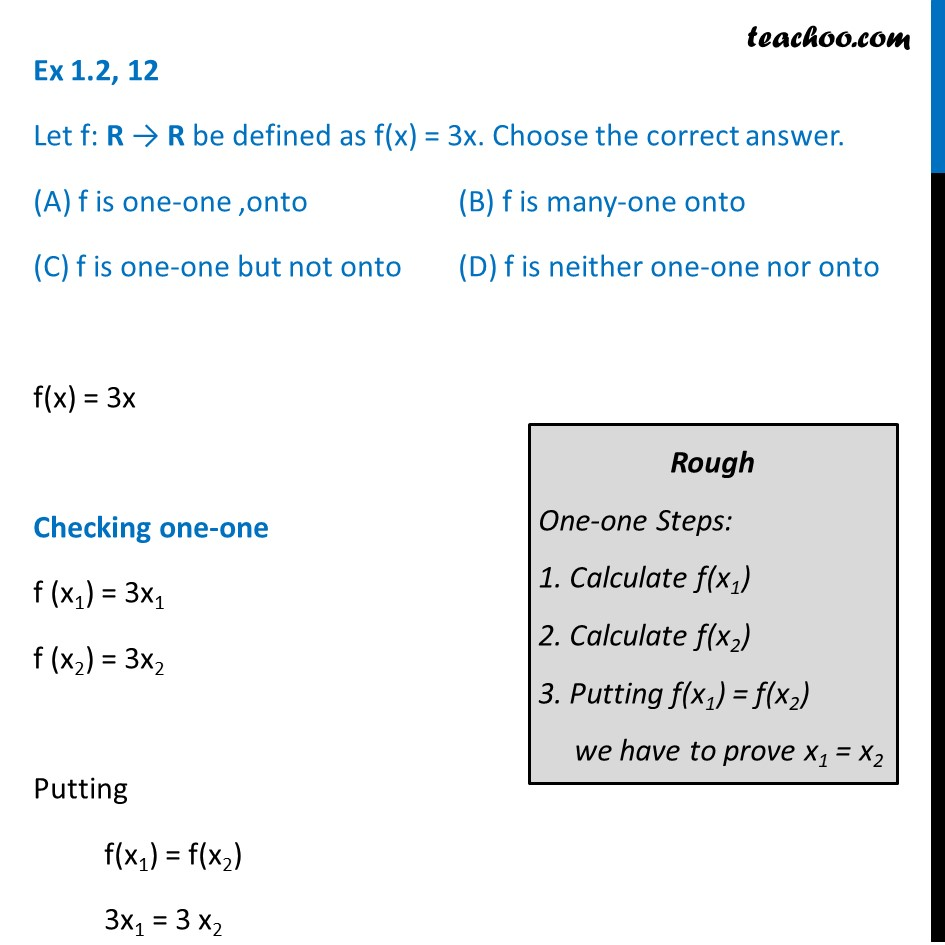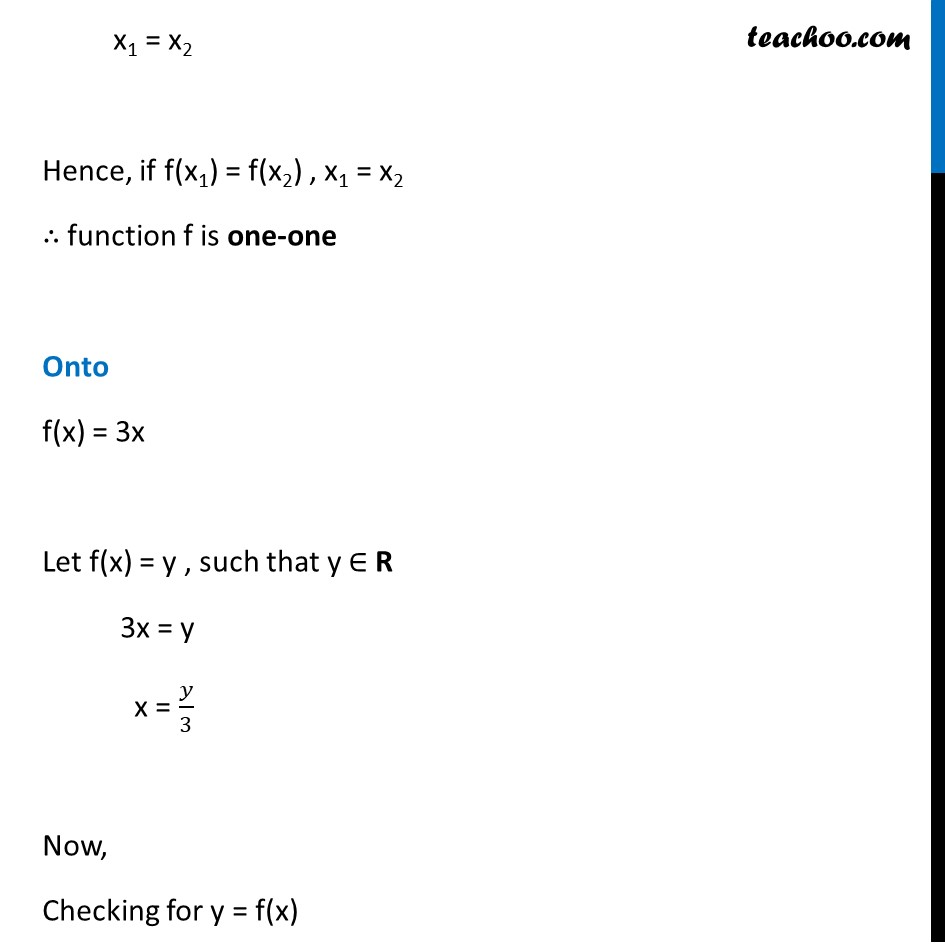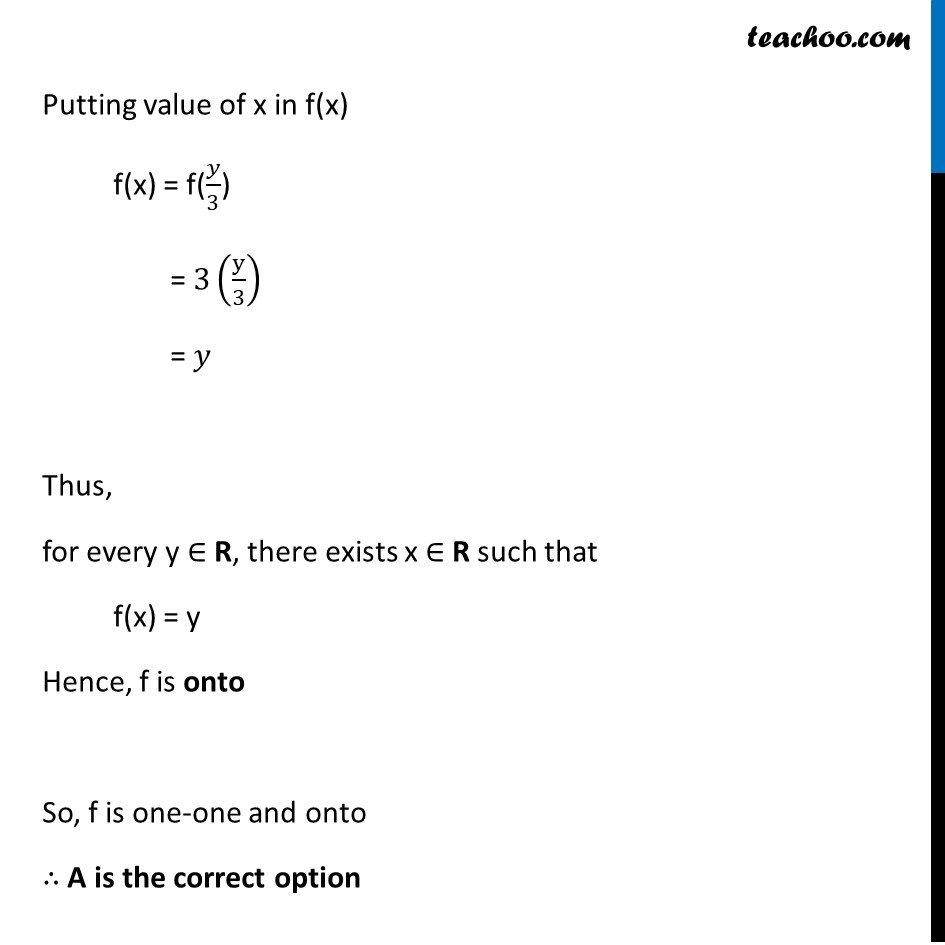To prove one-one & onto (injective, surjective, bijective)

Chapter 1 Class 12 Relation and Functions
Concept wiseLearn in your speed, with individual attention - Teachoo Maths 1-on-1 Class

### Transcript

Ex 1.2, 12 Let f: R → R be defined as f(x) = 3x. Choose the correct answer. (A) f is one-one ,onto (B) f is many-one onto (C) f is one-one but not onto (D) f is neither one-one nor onto f(x) = 3x Checking one-one f (x1) = 3x1 f (x2) = 3x2 Putting f(x1) = f(x2) 3x1 = 3 x2 Rough One-one Steps: 1. Calculate f(x1) 2. Calculate f(x2) 3. Putting f(x1) = f(x2) we have to prove x1 = x2 x1 = x2 Hence, if f(x1) = f(x2) , x1 = x2 ∴ function f is one-one Onto f(x) = 3x Let f(x) = y , such that y ∈ R 3x = y x = 𝑦/3 Now, Checking for y = f(x) Putting value of x in f(x) f(x) = f(𝑦/3) = 3(y/3) = 𝑦 Thus, for every y ∈ R, there exists x ∈ R such that f(x) = y Hence, f is onto So, f is one-one and onto ∴ A is the correct option